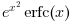Scilab 5.5.2
Change language to:
English - Français - 日本語 - Português -

See the recommended documentation of this function

Справка Scilab >> Special Functions > erfcx

# erfcx

scaled complementary error function.

### Calling Sequence

`y = erfcx(x)`

### Arguments

x

vector or matrix

y

vector or matrix (of same size than x)

### Description

Compute the scaled complementary error function of x, defined by. Note also thatcomputes the Faddeeva function w(x).

### Examples

```x = [1+2*%i,-1+2*%i,1e-6+2e-6*%i,0+2*%i];
erfcx(x)
// Equivalent to
exp (x.^2) .* erfc(x)```

### Algorithms

This function is based on the Faddeeva package library.